Custom SearchDensity and Specific Gravity

The density of a substance is its weight per unit volume. The unit volume in the English system of measurement is 1 cubic foot. In the metric system it is the cubic centimeter; therefore, density is expressed in pounds per cubic foot or in grams per cubic centimeter.

To find the density of a substance, you must know its weight and volume. You then divide its weight by its volume to find the weight per unit volume. In equation form, this is written as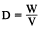Equation 2-4.

EXAMPLE: The liquid that fills a certain container weighs 1,497.6 pounds. The container is 4 feet long, 3 feet wide, and 2 feet deep. Its volume is 24 cubic feet (4 ft x 3 ft x 2 ft). If 24 cubic feet of this liquid weighs 1,497.6 pounds, then 1 cubic foot weighs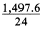or 62.4 pounds. Therefore, the density of the liquid is 62.4 pounds per cubic foot. This is the density of water at 4C and is usually used as the standard for comparing densities of other substances. The temperature of 4C was selected because water has its maximum density at this temperature. In the metric system, the density of water is 1 gram per cubic centimeter. The standard temperature of 4C is used whenever the density of liquids and solids is measured. Changes in temperature will not change the weight of a substance but will change the volume of the substance by expansion or contraction, thus changing the weight per unit volume.

In physics, the word specific implies a ratio. Weight is the measure of the earths attraction for a body. The earths attraction for a body is called gravity. Thus, the ratio of the weight of a unit volume of some substance to the weight of an equal volume of a standard substance, measured under standard pressure and temperature con-ditions, is called specific gravity. The terms specific weight and specific density are sometimes used to express this ratio.

The following formulas are used to find the specific gravity (sp gr) of solids and liquids, with water used as the standard substance.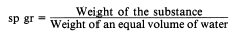or,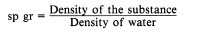The same formulas are used to find the specific gravity of gases by substituting air, oxygen, or hydrogen for water.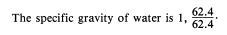If a cubic foot of a certain liquid weighs 68.64 pounds, then its specific gravity is 1.1,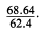Thus, the specific gravity of the liquid is the ratio of its density to the density of water. If the specific gravity of a liquid or solid is known, the density of the liquid or solid maybe obtained by multiplying its specific gravity by the density of water. For example, if a certain hydraulic liquid has a specific gravity of 0.8, 1 cubic foot of the liquid weighs 0.8 times as much as a cubic foot of water0.8 times 62.4, or 49.92 pounds. In the metric system, 1 cubic centimeter of a substance with a specific gravity of 0.8 weighs 1 times 0.8, or 0.8 grams. (Note that in the metric system the specific gravity of a liquid or solid has the same numerical value as its density, because water weighs 1 gram per cubic centimeter.)

Specific gravity and density are independent of the size of the sample under consideration and depend only on the substance of which it is made. A device called a hydrometer is used for measuring the specific gravity of liquids.

Pascals Law

Recall from chapter 1 that the foundation of modern hydraulics was established when Pascal discovered that pressure in a fluid acts equally in all directions. This pressure acts at right angles to the containing surfaces. If some type of pressure gauge, with an exposed face, is placed beneath the surface of a liquid (fig. 2-6) at a specific depth and pointed in different directions, the pressure will read the same. Thus, we can say that pressure in a liquid is independent of direction.

Pressure due to the weight of a liquid, at any level, depends on the depth of the fluid from the surface. If the exposed face of the pressure gauges, figure 2-6, are moved closer to the surface of the liquid, the indicated pressure will be less. When the depth is doubled, the indicated pressure is doubled. Thus the pressure in a liquid is directly proportional to the depth.

Consider a container with vertical sides (fig. 2-7) that is 1 foot long and 1 foot wide. Let it be filled with water 1 foot deep, providing 1 cubic foot of water. We learned earlier in this chapter that 1 cubic foot of water weighs 62.4 pounds. Using this information and equation 2-2, P = F/A, we can calculate the pressure on the bottom of the container.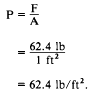Since there are 144 square inches in 1 square foot,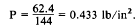This can be stated as follows: the weight of a column of water 1 foot high, having a cross-sectional area of 1 square inch, is 0.433 pound. If the depth of the column is tripled, the weight of the column will be 3 x 0.433, or 1.299 pounds, and the pressure at the bottom will be 1.299 lb/in2 (psi), since pressure equals the force divided by the area. Thus, the pressure at any depth in a liquid is equal to the weight of the column of liquid at that depth divided by the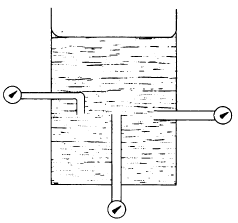Figure 2-6.Pressure of a liquid is independent of direction.

cross-sectional area of the column at that depth. The volume of a liquid that produces the pressure is referred to as the fluid head of the liquid. The pressure of a liquid due to its fluid head is also dependent on the density of the liquid.

If we let A equal any cross-sectional area of a liquid column and h equal the depth of the column, the volume becomes Ah. Using equation 2-4, D = W/V, the weight of the liquid above area A is equal to AhD.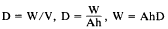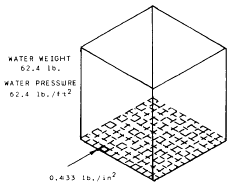Figure 2-7.Water pressure in a 1-cubic-foot container.

Since pressure is equal to the force per unit area, set A equal to 1. Then the formula pressure becomes

P =hD Equation 2-5.

It is essential that h and D be expressed in similar units. That is, if D is expressed in pounds per cubic foot, the value of h must be expressed in feet. If the desired pressure is to be expressed in pounds per square inch, the pressure formula, equation 2-5, becomes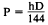Equation 2-6.Integrated Publishing, Inc. - A (SDVOSB) Service Disabled Veteran Owned Small Business